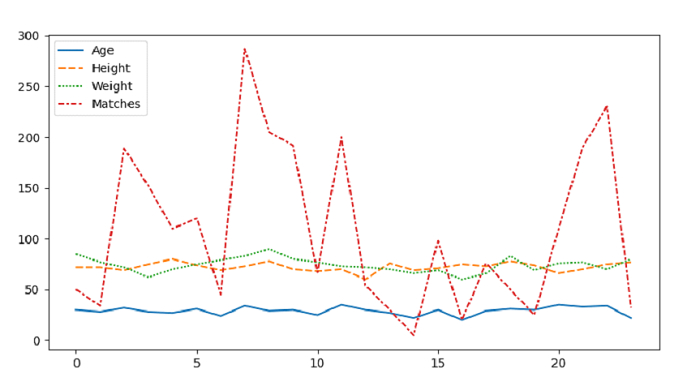# Draw a lineplot passing the entire dataset with Seaborn â€“ Python Pandas

Lineplot in Seaborn is used to draw a line plot with possibility of several semantic groupings. The seaborn.lineplot() is used for this. To plot lineplot with entire dataset, simply use the lineplot() and set the complete dataset in it without mentioning the x and y values.

Let’s say the following is our dataset in the form of a CSV file − Cricketers2.csv

At first, import the required libraries −

import seaborn as sb
import pandas as pd
import matplotlib.pyplot as plt

Load data from a CSV file into a Pandas DataFrame −

dataFrame = pd.read_csv("C:\Users\amit_\Desktop\Cricketers2.csv")

Plot lineplot with entire dataset −

sb.lineplot(data=dataFrame)



## Example

Following is the code −

import seaborn as sb
import pandas as pd
import matplotlib.pyplot as plt

# Load data from a CSV file into a Pandas DataFrame

# plot lineplot with entire dataset
sb.lineplot(data=dataFrame)

plt.show()

## Output

This will produce the following output −Updated on: 28-Sep-2021

95 Views